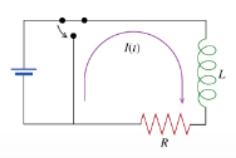# Problem: To understand the mathematics of current decay in an L-R circuit.A DC voltage source is connected to a resistor of resistance R and an inductor with inductance L, forming the circuit shown in the figure. For a long time before t = 0, the switch has been in the position shown, so that a current I0 has been built up in the circuit by the voltage source. At t = 0 the switch is thrown to remove the voltage source from the circuit. This problem concerns the behavior of the current from the circuit. This problem concerns the behavior of the current I (t) through the inductor and the voltage V (t) across the indicator at time t after t = 0.Part A. What is the differential equation satisfied by the current I (t) after time t = 0? Express dI(t)/dt in terms of I (t), R, and L.Part B. What is the expression for I(t) obtained by solving the differential equation that I(t) satisfied after t = 0? Express your answer in terms f the initial current I0 as well as L, R, and t.

###### FREE Expert Solution

As electric current flows in an inductor, energy is stored in the inductor.

This energy is expressed by:

$\overline{){\mathbf{I}}{\mathbf{\left(}}{\mathbf{t}}{\mathbf{\right)}}{\mathbf{R}}{\mathbf{+}}{\mathbf{L}}\frac{\mathbf{d}\mathbf{I}\mathbf{\left(}\mathbf{t}\mathbf{\right)}}{\mathbf{d}\mathbf{t}}{\mathbf{=}}{\mathbf{0}}}$

Part A

From the equation of the energy stored in a conductor, we can solve for the differential, dI(t)/dt as:

84% (3 ratings)###### Problem Details

To understand the mathematics of current decay in an L-R circuit.A DC voltage source is connected to a resistor of resistance R and an inductor with inductance L, forming the circuit shown in the figure. For a long time before t = 0, the switch has been in the position shown, so that a current I0 has been built up in the circuit by the voltage source. At t = 0 the switch is thrown to remove the voltage source from the circuit. This problem concerns the behavior of the current from the circuit. This problem concerns the behavior of the current I (t) through the inductor and the voltage V (t) across the indicator at time t after t = 0.

Part A. What is the differential equation satisfied by the current I (t) after time t = 0? Express dI(t)/dt in terms of I (t), R, and L.

Part B. What is the expression for I(t) obtained by solving the differential equation that I(t) satisfied after t = 0? Express your answer in terms f the initial current I0 as well as L, R, and t.

Frequently Asked Questions

What scientific concept do you need to know in order to solve this problem?

Our tutors have indicated that to solve this problem you will need to apply the LR Circuits concept. You can view video lessons to learn LR Circuits. Or if you need more LR Circuits practice, you can also practice LR Circuits practice problems.